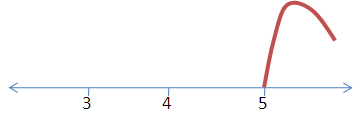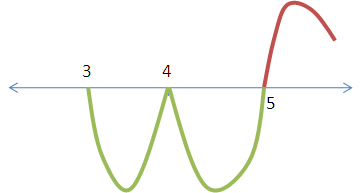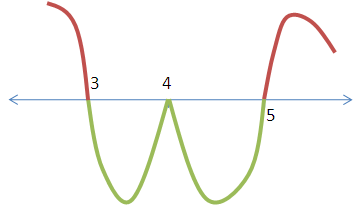Click to Chat

1800-1023-196

+91-120-4616500

CART 0

• 0

MY CART (5)

Use Coupon: CART20 and get 20% off on all online Study Material

ITEM
DETAILS
MRP
DISCOUNT
FINAL PRICE
Total Price: Rs.

There are no items in this cart.
Continue Shopping```Quadratic Equation and Expressions Study Material for IIT JEE

Table of Content

Polynomial Equation of Degree n

Descarte’s Rule of Signs

Sturm Theorem

The Wave Curve MethodQuadratic Equations constitute an important part of Algebra. This portion lays the foundation of various important topics and hence it is becomes vital to master the topic. It is a comparatively simple topic and with a bit of hard work it becomes easy to fetch the questions of this topic in the IIT JEE. The various heads covered under this topic include:

Basic Concepts

Polynomial Equation of Degree n

The Method of Intervals Wavy Curve Method

Interval in which the Roots Lie

Maximum And Minimum Value of a Quadratic Expression

Resolution of a Quadratic Function Into Linear Factors

The word quadratic equation is derived from the Latin word ‘quadratus’ meaning a square. A quadratic equation is any equation having the form
ax2+bx+c =0,
where x represents an unknown, and a, b, and c are constants with a not equal to 0. If a = 0, then the equation is linear, not quadratic. The constants a, b, and c are called the coefficients. While ‘c’ represents the constant term, b is the linear coefficient and ‘a’ the quadratic coefficient. The quadratic equations involve only one unknown and hence are called univariate. The quadratic equations are basically polynomial equations since they contain non-integral powers of x. Since the greatest power is two so they are second degree polynomial equations.

We shall discuss the terms associated with quadratic equations here in brief as they have been discussed in detail in the coming sections.

Discriminant of a Quadratic Equation: The discriminant of a quadratic equation is defined as the number D= b2-4ac and is determined form the coefficients of the equation ax2+bx+c =0. The discriminant reveals the nature of roots an equation has.

Note: b2 – 4ac is derived from the quadratic formula

The below table lists the different types of roots associated with the values of determinant.

Discriminant                                         Roots

D < 0                                        two roots which are complex conjugates

D = 0                                        one real root of multiplicity two

D > 0                                        two distinct real roots

D = positive perfect square          two distinct rational roots (assumes a, b and c are rational

Example: Consider the quadratic equation y = 3x2+9x+5. Find its discriminant.

Solution: The given quadratic equation is y = 3x2+9x+5.

The formula of discriminant is D = b2-4ac.

Hence, here a= 3, b= 9 and c= 5 and so the discriminant is given by

D = 92-4.3.5 = 31.

You may refer the Sample Papers to get an idea about the types of questions asked.

Polynomial Equation of Degree n

A polynomial equation is an equation that can be written in the form

axn + bxn-1 + . . . + rx + s = 0,
where a, b, . . . , r and s are constants. The largest exponent of x appearing in a non-zero term of a polynomial is called the degree of that polynomial.

Examples:

Consider the equation 3x+1 = 0. The equation has degree 1 as the largest power of x that appears in the equation is 1. Such equations are called linear equations.

x2 +x-3 = 0 has degree 2 since this is the largest power of x. such degree 2 equations are called quadratic equations or simply quadratics.

Degree 3 equations like x3+2x2-4=0 are called cubic.

A polynomial equation of degree n has n roots, but some of them may be multiple roots. For example, consider x3- 9x2+24x-16 =0.
It is clearly a polynomial of degree 3 and so will have three roots. The equation can be factored as (x-1) (x-4) (x-4) =0. Hence, this implies that the roots of the equation are x=1, x=4, x=4. Hence, the root x=4 is repeated.

Descarte’s Rule of Signs

This is a very famous rule that helps in getting an idea about the roots of a polynomial equation. The rule states that the number of positive real roots of Pn(x) = 0 cannot be more than the number of sign changes. Similarly, the number of negative roots cannot be more than the number of sign changes in Pn(-x).

For instance, consider the equation P5(x) = x5+2x4-x3+x2-x+2 = 0.
Now as stated above, since this equation has four sign changes so it can have at most four positive real roots. Now if we see P5(-x), it has only one sign change as
P5(-x) = -x5+2x4+x3+x2+x+2 = 0

Hence, it can have only one negative real root.

Watch this Video for more reference

Remark: The Descarte’s rule only gives an idea about the maximum number of positive or negative real roots but cannot give the exact number. For getting an idea on the exact number of roots, the Sturm’s Theorem should be used.

Sturm Theorem

This theorem states that the number of real roots of the equation f(x) = 0 at [a, b] equals the difference between the number of sign changes in the Sturm sequence at x = a and x= b, provided that f (a) ≠ 0 and f (b) ≠ 0.
We know that any nth degree polynomial has exactly n roots. So, it is obvious that the number of complex roots equals total roots – number of real roots. Hence,

No. of complex roots = n - no. of real roots, where a real root of multiplicity r is to be counted r times. In case, the coefficient of the polynomial are real, the complex roots are α ± iβ and hence total no of complex roots are even.

The Wave Curve Method

Here we shall discuss an example to explain this method, for theory you can refer the coming sections.
Consider the question (x-3) (x-4)2 (x-5)3 = 0.
First step should be to put each factor equal to zero. Here, we get

(x-3) = 0, (x-4)2 = 0, (x-5)3 = 0

By equating all terms equal to zero, we obtain various values of x which are called the critical points. Hence, here 3, 4, 5 are the critical points.
Now plot these points on a number line in increasing order. Once you plot the points, put a value greater than the rightmost point in the entire function.
Here, the rightmost point is 5. So put a value greater than 5, say 6 in the entire function. On putting x=6 in the entire function we get a positive sign so we start drawing the curve above the number line.
Now comes the important step. Check for the exponents of each term. If the power of the factor is odd, you must change the side of the wavy curve ( if above then below and vice versa. Otherwise, you must continue with the same side of the number line. We explain this step for this example:First on putting the value 6 we get a positive sign so draw the curve on the above side of axis.Now we reach 5. We have obtained 5 from the factor (x-5)3. The exponent of this factor is odd, so we need to change the side of the curve. Again, for the next factor  (x-4)2, the power is even and so we have to retain the side of the wavy curve. It was below the axis so even it’ll remain there only. The curve looks like thisNext factor is (x-3). So its power is 1, again odd. Again change the side of the curve. Initially, it was below, now it will be above the line.Hence this is how the curve looks like. It is clear that the function is positive in the intervals when the curve lies above the axis and negative in the intervals where the curve lies below the axis.

To read more, Buy study materials of Quadratic Equation comprising study notes, revision notes, video lectures, previous year solved questions etc. Also browse for more study materials on Mathematics here.
```### Course Features

• 731 Video Lectures
• Revision Notes
• Previous Year Papers
• Mind Map
• Study Planner
• NCERT Solutions
• Discussion Forum
• Test paper with Video Solution